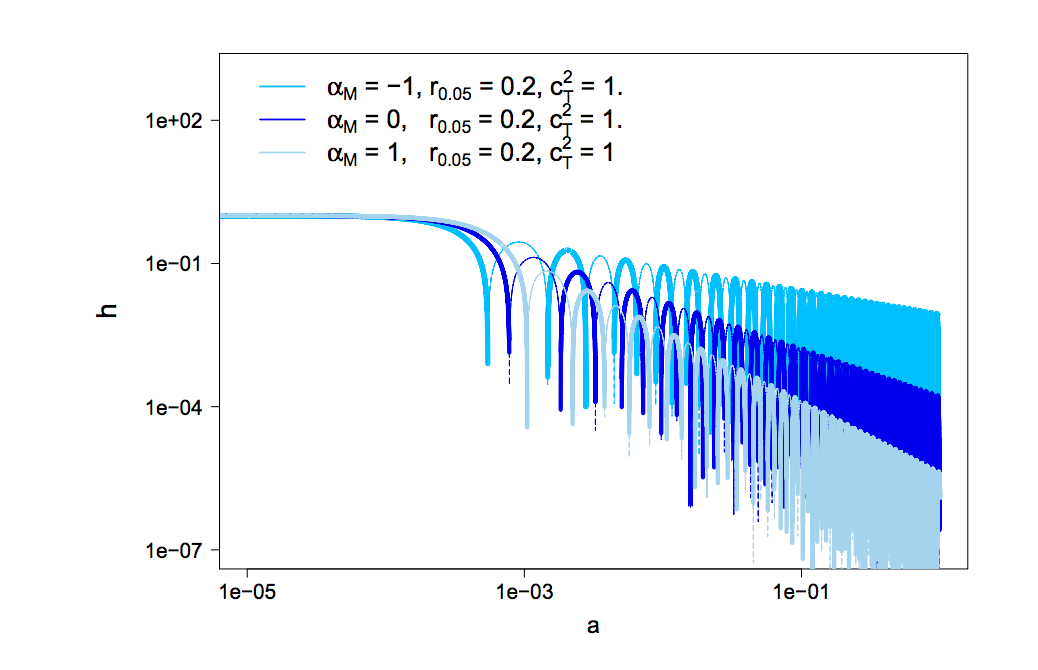# Friction in Gravitational Waves: a test for early-time modified gravityAuthors: Pettorino, V., Amendola, L. Journal: Physics Letters B Year: 2015 Download: ADS | arXiv

## Abstract

Modified gravity theories predict in general a non standard equation for the propagation of gravitational waves. Here we discuss the impact of modified friction and speed of tensor modes on cosmic microwave polarization B modes. We show that the non standard friction term, parametrized by αM, is degenerate with the tensor-to-scalar ratio r, so that small values of r can be compensated by negative constant values of αM. We quantify this degeneracy and its dependence on the epoch at which αM is different from the standard, zero, value and on the speed of gravitational waves cT. In the particular case of scalar-tensor theories, αM is constant and strongly constrained by background and scalar perturbations, 0≤αM<0.01 and the degeneracy with r is removed. In more general cases however such tight bounds are weakened and the B modes can provide useful constraints on early-time modified gravity.